Preliminary Spatial Data Analysis

A semivariance analysis of the coal seam thickness thick data set is performed in Getting Started: VARIOGRAM Procedure of the VARIOGRAM procedure. The analysis considers the spatial random field (SRF)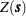of the Thick variable to be free of surface trends. The expected value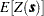is then a constant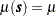, which suggests that you can work with the original thickness data rather than residuals from a trend surface fit. In fact, a reasonable approximation of the spatial process generating the coal seam data is given by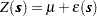where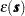is a Gaussian SRF with Gaussian covariance structure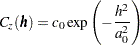Of note, the term "Gaussian" is used in two ways in this description. For a set of locations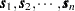, the random vector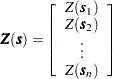has a multivariate Gaussian or normal distribution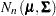. The (,)th element of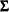is computed by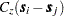, which happens to be a Gaussian functional form.

Any functional form for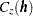that yields a valid covariance matrixcan be used. Both the functional form ofand the parameter values

1.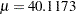2.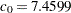3.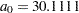are estimated by using PROC VARIOGRAM in section Theoretical Semivariogram Model Fitting in the VARIOGRAM procedure. Specifically, the expected valueis reported in the VARIOGRAM procedure OUTV output data set, and the parameters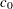and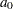are estimates derived from a weighted least squares fit.

The choice of a Gaussian functional form foris simply based on the data, and it is not at all crucial to the simulation. However, it is crucial to the simulation method used in PROC SIM2D thatbe a Gaussian SRF. For details, see the section Computational and Theoretical Details of Spatial Simulation.